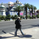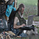TradingView
533 views
Same as the overlay version, without the leading / price lines, as an indicator.
Open-source script

In true TradingView spirit, the author of this script has published it open-source, so traders can understand and verify it. Cheers to the author! You may use it for free, but reuse of this code in a publication is governed by House Rules. You can favorite it to use it on a chart.

Want to use this script on a chart?
```study("Ichimoku_on_steroids v 1.0 IN")

varLo = input(title="Fast Leading Line", type=integer, defval=9, minval=1, maxval=99999)
varHi = input(title="Slow Leading Line", type=integer, defval=26, minval=1, maxval=99999)
emafreq = input(title="Ema on price frequency", type=integer, defval=10, minval=1, maxval=99999)

a = lowest(varLo)
b = highest(varLo)
c = (a + b ) / 2

d = lowest(varHi)
e = highest(varHi)
f = (d + e) / 2

g = ((c + f) / 2)[varHi]
h = ((highest(varHi * 2) + lowest(varHi * 2)) / 2)[varHi]

z = ema(close, emafreq)

bgcolor(z > h and z > g ? green : z < h and z < g ? red : yellow, transp=70)```

## Commentsnikakolin
study("Ichimoku_on_steroids v 1.0 IN")

varLo = input(title="Fast Leading Line", type=integer, defval=9, minval=1, maxval=99999)
varHi = input(title="Slow Leading Line", type=integer, defval=26, minval=1, maxval=99999)
emafreq = input(title="Ema on price frequency", type=integer, defval=10, minval=1, maxval=99999)

a = lowest(varLo)
b = highest(varLo)
c = (a + b ) / 2

d = lowest(varHi)
e = highest(varHi)
f = (d + e) / 2

g = ((c + f) / 2)
h = ((highest(varHi * 2) + lowest(varHi * 2)) / 2)

z = ema(close, emafreq)

bgcolor(z > h and z > g ? green : z < h and z < g ? red : yellow, transp=70)
Replynikakolin
to remove the displacement, all you need to do is remove the from the code :)
Replyjamc
hm ... remove the 'squarebracket' varHi 'squarebracket' ...
Reply
Profile Profile Settings Account and Billing Referred friends Coins My Support Tickets Help Center Private Messages Chat Sign Out